A quadratic programming problem is a mathematical programming problem, which consists of an objective function composed of linear and quadratic terms and a set of linear constraints. A quadratic programming problem can be solved by a modification of the simplex procedure.

Quadratic programming model is the simplest nonlinear extension of the linear programming model.

The general quadratic programming problem is given by

Maximize f =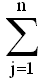cjxj +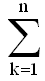cjkxjxk

subject toaijxj - bi ≤ 0 , i = 1, 2, ...., m

xj ≥ 0, j = 1, 2, ...., n

If the quadratic form is negative semidefinite and symmetric, then it is a concave function of the decision variables. Since the constraints are linear, the feasible region is convex. Thus, Kuhn-Tucker conditions in this case are both necessary and sufficient.

To derive these conditions, we use multipliers λi ( i = 1, 2, ...., m) corresponding to the constraintsaijxj - bi ≤ 0;
and μj ( j = 1, 2, ...., n) corresponding to the non-negativity constraints.

df/dxj = cj + 2cjkxk , j = 1, 2, ...., n

On small screens, use horizontal scrollbar to view full table

 d ---- dxj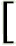aijxj - bi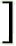= aij ; i = 1, 2, ...., m; j = 1, 2, ...., n

Let Si = bi -aijxj; i = 1, 2, ...., m .....(i)
Where Si is a slack variable.

Hence, Kuhn-Tucker conditions are given by

cj + 2cjkxk -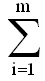λiaij + μj = 0; j = 1, 2, ...., n

or - 2cjkxk +λiaij - μj = cj; j = 1, 2, ...., n .....(ii)

Siλi = 0, xjμj = 0
Si, λi, μj, xj ≥ 0, i = 1, 2, ...., m; j = 1, 2, ...., n

If we ensure that the pairs ( λi, Si) and (μj, xj) are not into the basis simultaneously, then the conditions Siλi = 0 and xjμj = 0 will be automatically satisfied. Therefore, we use simplex procedure with a restricted entry rule.Don't be too concerned about this horrendous notation. The next section provides an example that will make the concepts clear. So you can easily skip this section and move to the next example.

Share and Recommend

•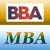MBA & BBA
•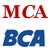BCA & MCA
•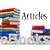Ebooks & Articles
•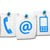Contact us